### IMO Shortlist 2012 problem C1

Kvaliteta:
Avg: 3,5
Težina:
Avg: 6,0
Several positive integers are written in a row. Iteratively, Alice chooses two adjacent numbers$x$ and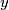$y$ such that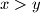$x>y$ and$x$ is to the left of$y$, and replaces the pair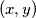$(x,y)$ by either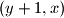$(y+1,x)$ or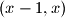$(x-1,x)$. Prove that she can perform only finitely many such iterations.

Proposed by Warut Suksompong, Thailand
Izvor: Međunarodna matematička olimpijada, shortlist 2012